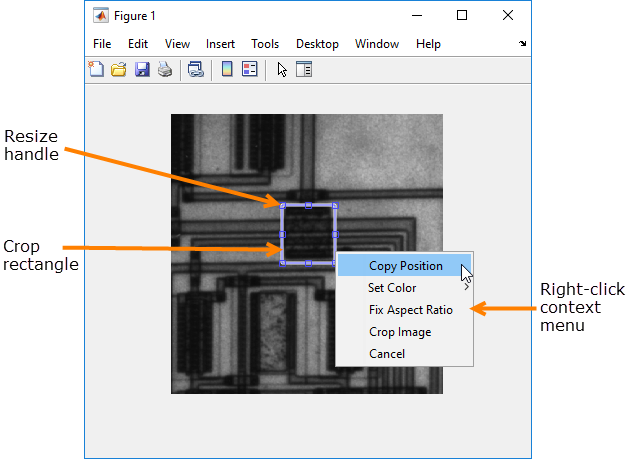# imcrop

## 语法

``Icropped = imcrop``
``Icropped = imcrop(I)``
``Xcropped = imcrop(X,cmap)``
``___ = imcrop(h)``
``Icropped = imcrop(I,rect)``
``Xcropped = imcrop(X,cmap,rect)``
``___ = imcrop(xref,yref,___)``
``[___,rectout] = imcrop(___)``
``````[xrefout,yrefout,___] = imcrop(___)``````
``imcrop(___)``

## 说明

### 以交互方式裁剪图像

``Icropped = imcrop` 创建与当前图窗中显示的灰度、真彩色或二值图像相关联的交互式裁剪图像工具。`imcrop` 返回裁剪的图像 `Icropped`。使用此语法和其他交互式语法，裁剪图像工具会阻止 MATLAB® 命令行，直到您完成操作。有关使用裁剪图像工具的详细信息，请参阅交互式行为。`

``Icropped = imcrop(I)` 在图窗窗口中显示灰度图像、真彩色图像或二值图像 `I`，并创建与该图像相关联的交互式裁剪图像工具。`
``Xcropped = imcrop(X,cmap)` 使用颜色图 `cmap` 在图窗中显示索引图像 `X`，并创建与该图像相关联的交互式裁剪图像工具。`imcrop` 返回裁剪的索引图像 `Xcropped`，该图像也具有颜色图 `cmap`。`
``___ = imcrop(h)` 创建与句柄 `h` 指定的图像相关联的交互式裁剪图像工具。`

### 通过指定裁剪区域裁剪图像

``Icropped = imcrop(I,rect)` 根据在裁剪矩形 `rect` 中指定的位置和维度裁剪图像 `I`。裁剪的图像包括输入图像中该矩形完全或部分包围的所有像素。输出图像的实际大小并不始终与 `rect` 指定的宽度和高度完全一致。例如，假设 `rect` 是 `[20 20 40 30]`（使用默认的空间坐标系）。指定矩形的左上角是空间 (x,y) 坐标为 (20,20) 的像素的中心。矩形的右下角是空间 (x,y) 坐标为 (60,50) 的像素的中心。生成的输出图像大小为 31×41 像素，而不是 30×40 像素。`

``Xcropped = imcrop(X,cmap,rect)` 根据裁剪矩形 `rect` 中指定的位置和维度，裁剪具有颜色图 `cmap` 的索引图像 `X`。`imcrop` 返回裁剪的索引图像 `Xcropped`，该图像也具有颜色图 `cmap`。`
``___ = imcrop(xref,yref,___)` 使用 `xref` 和 `yref` 定义的世界坐标系裁剪输入图像。在 `xref` 和 `yref` 输入参数后，您可以指定包含输入图像 `I` 或 `X` 的任何语法的参数。`

### 指定其他输出选项

``[___,rectout] = imcrop(___)` 还在 `rectout` 中返回裁剪矩形的位置。您可以使用任何其他语法的输入参数。`
``````[xrefout,yrefout,___] = imcrop(___)``` 还在 `xrefout` 和 `yrefout` 中返回输入图像的图像范围。```
`不带输出参数的 `imcrop(___)` 在新图窗窗口中显示裁剪的图像。此语法不支持分类图像。`

## 示例

`I = imread('cameraman.tif');`

`[J,rect] = imcrop(I);``whos`
```Name Size Bytes Class Attributes I 256x256 65536 uint8 J 121x126 15246 uint8 rect 1x4 32 double ```

`I = imread('circuit.tif');`

`I2 = imcrop(I,[75 68 130 112]);`

```subplot(1,2,1) imshow(I) title('Original Image') subplot(1,2,2) imshow(I2) title('Cropped Image')``````I = imread('parkavenue.jpg'); imshow(I)````targetSize = [300 600];`

`r = centerCropWindow2d(size(I),targetSize);`

```J = imcrop(I,r); imshow(J)````load trees`

`X2 = imcrop(X,map,[30 30 50 75]);`

```subplot(1,2,1) imshow(X,map) title('Original Image') subplot(1,2,2) imshow(X2,map) title('Cropped Image')```## 输入参数

• 表示灰度图像的 m×n 数值矩阵

• 表示真彩色图像的 m×n×3 数值数组

• 表示二值掩膜的 m×n 逻辑矩阵。

• 表示标签图像的 m×n 分类矩阵。

注意

对于分类输入，您必须指定裁剪矩形 `rect`。交互式语法不支持分类输入。

## 输出参数

• 如果指定输入图像 `I`，则输出图像与输入图像具有相同的数据类型。

• 如果不指定输入图像，则输出图像通常与输入图像具有相同的数据类型。但是，如果输入图像具有数据类型 `int16``single`，则输出图像具有数据类型 `double`

## 详细信息

### 交互式行为BackspaceEscDelete，或在裁剪矩形内右键点击，然后从上下文菜单中选择取消